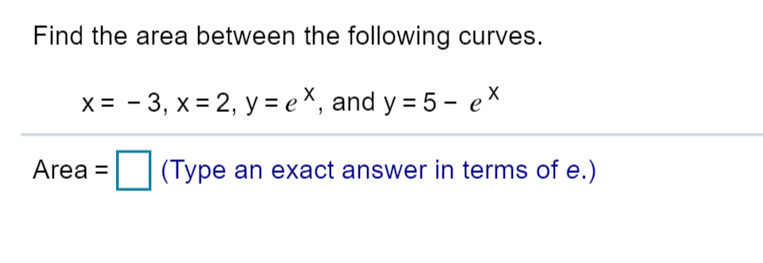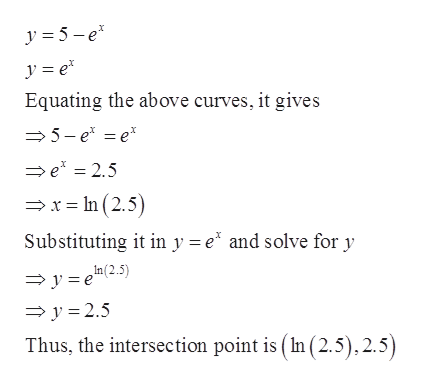# Find the area between the following curves.= -3, x 2, y ex, and y = 5 - exArea(Type an exact answer in terms of e.)

Question
1 viewshelp_outlineImage TranscriptioncloseFind the area between the following curves. = -3, x 2, y ex, and y = 5 - ex Area (Type an exact answer in terms of e.) fullscreen
check_circle

Step 1

To find the area bounded by the following curves.

Step 2

The graph of given curves is shown below. The bounded regions intersect at one point.

Step 3

Find the intersect point by eq...help_outlineImage Transcriptionclosey 5e y e Equating the above curves, it gives 5-e e e = 2.5 x =In (2.5) Substituting it in y e and solve for y In (2.5) y e y 2.5 Thus, the intersection point is (In (2.5),2.5) fullscreen

### Want to see the full answer?

See Solution

#### Want to see this answer and more?

Solutions are written by subject experts who are available 24/7. Questions are typically answered within 1 hour.*

See Solution
*Response times may vary by subject and question.
Tagged in

### Calculus